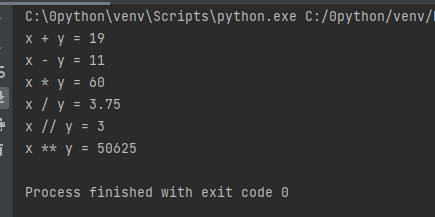# Python操作符（运算符）

## 1.什么是python运算符？

``print(eval('2+3'))``

``5``

## 2.算术运算符

+ 12.45 + 15 27.45
4.56 – 0.26 4.3
* 5 * 3.6 18.0
/ 除法（和数学中的规则一样） 7 / 2 3.5
// 整除（只保留商的整数部分） 7 // 2 3
% 取余，即返回除法的余数 （取模运算符） 7 % 2 1
** 幂运算/次方运算，即返回 x 的 y 次方 2 ** 4 16，即 24

``````x = 15
y = 4

# Output: x + y = 19
print('x + y =',x+y)

# Output: x - y = 11
print('x - y =',x-y)

# Output: x * y = 60
print('x * y =',x*y)

# Output: x / y = 3.75
print('x / y =',x/y)

# Output: x // y = 3
print('x // y =',x//y)

# Output: x ** y = 50625
print('x ** y =',x**y)``````## Python比较运算符

== 等于 – 比较对象是否相等 (a == b) 返回 False。
!= 不等于 – 比较两个对象是否不相等 (a != b) 返回 true.
> 大于 – 返回x是否大于y (a > b) 返回 False。
< 小于 – 返回x是否小于y。所有比较运算符返回1表示真，返回0表示假。这分别与特殊的变量True和False等价。 (a < b) 返回 true。
>= 大于等于 – 返回x是否大于等于y。 (a >= b) 返回 False。
<= 小于等于 – 返回x是否小于等于y。 (a <= b) 返回 true。

``````x = 10
y = 12
print('x=',x)
print('y=',y)
# Output: x > y is False
print('x > y is',x>y)

# Output: x < y is True
print('x < y is',x<y)

# Output: x == y is False
print('x == y is',x==y)

# Output: x != y is True
print('x != y is',x!=y)

# Output: x >= y is False
print('x >= y is',x>=y)

# Output: x <= y is True
print('x <= y is',x<=y)``````
`结果`

## 4.逻辑运算符

Python 的逻辑运算符主要包括 and（逻辑与）、or（逻辑或）以及 not（逻辑非）。

Python逻辑运算符语法

and 逻辑与（简称“与”） a and b 只有操作数 a 和 操作数 b 都是 True 时，才返回 True，否则返回 False。
or 逻辑或（简称“或”） a or b 只要操作数中有一个为 True，则返回 True，否则返回 False。
not 逻辑非（简称“非”） not a 如果操作数 a 为 True，则返回 False。如果操作数 a 为 False，则返回 True。

### 例4.1 python逻辑运算符范例

``````x = True
y = False

print('x and y is',x and y)
print('x or y is',x or y)
print('not x is',not x)``````
`结果`
``````x and y is False
x or y is True
not x is False``````

### 以下是truth table

The truth table for `and` is given below:

Truth table for `and`
A B A and B
True True True
True False False
False True False
False False False

`or` will result into `True` if any of the operands is `True`. The truth table for `or` is given below:

Truth table for `or`
A B A or B
True True True
True False True
False True True
False False False

`not` operator is used to invert the truth value. The truth table for `not` is given below:

Truth tabel for `not`
A not A
True False
False True

## 5.按位操作符

2的二进制是10
7的二进制是111

Operator Meaning Example
> x=0000 1010
> y=0000 0100
& AND x & y = 0 (`0000 0000`)
|  OR x | y = 14 (`0000 1110`)
~  NOT ~x = -11 (`1111 0101`)
^  XOR x ^ y = 14 (`0000 1110`)
>>  按位右移 x >> 2 = 2 (`0000 0010`)
<< 按位左移 x << 2 = 40 (`0010 1000`)

## 6.赋值运算符

Python 中使用赋值运算符为变量赋值。

a = 5 是一个简单的赋值运算符，它将右侧的值 5 分配给左侧的变量 a。

Python 中有各种复合运算符，例如 += 5，等价于 a = a + 5。

= x = 5 x = 5
+= x += 5 x = x + 5
-= x -= 5 x = x – 5
*= x *= 5 x = x * 5
/= x /= 5 x = x / 5
%= x %= 5 x = x % 5
//= x //= 5 x = x // 5
**= x **= 5 x = x ** 5
&= x &= 5 x = x & 5
|= x |= 5 x = x | 5
^= x ^= 5 x = x ^ 5
>>= x >>= 5 x = x >> 5
<<= x <<= 5 x = x << 5

## 7.特殊运算符

Python 语言提供了一些特殊类型的运算符，例如身份运算符或成员运算符。

### 7.1身份运算符

is 和 is not 是 Python 中的身份运算符。它们用于检查两个值（或变量）是否位于内存的同一部分。两个变量相等并不意味着它们是相同的。

is is 是判断两个操作数是否相同（引用同一个对象） x is True
is not is not 是判断两个操作数不同 （引用不同对象） x is not True

``````x1 = 5
y1 = 5
x2 = 'Hello'
y2 = 'Hello'
x3 = [1,2,3]
y3 = [1,2,3]

# Output: False
print(x1 is not y1)

# Output: True
print(x2 is y2)

# Output: False
print(x3 is y3)``````

``````False
True
False``````

### 7.2成员运算符

in 和 not in 是 Python 中的成员运算符。它们用于测试值或变量是否在序列（字符串、列表、元组、集合和字典）中。

in 如果在指定的序列中找到值返回 True，否则返回 False。 x 在 y 序列中 , 如果 x 在 y 序列中返回 True。
not in 如果在指定的序列中没有找到值返回 True，否则返回 False。 x 不在 y 序列中 , 如果 x 不在 y 序列中返回 True。

``````x = 'Hello world'
y = {1:'a',2:'b'}

# Output: True
print('H' in x)

# Output: True
print('hello' not in x)

# Output: True
print(1 in y)

# Output: False
print('a' in y)``````
`结果`
``````True
True
True
False``````
```这里，“H”在 x 中，但“hello”在 x 中不存在（请记住，Python 区分大小写）。

```

Posted in Python教程